# Collapse multiple Columns in Pandas

While operating dataframes in Pandas, we might encounter a situation to collapse the columns. Let it be
cumulated data of multiple columns or collapse based on some other requirement. Let’s see how to collapse multiple columns in Pandas.

Following steps are to be followed to collapse multiple columns in Pandas:

Step #1: Load numpy and Pandas.
Step #2: Create random data and use them to create a pandas dataframe.
Step #3: Convert multiple lists into a single data frame, by creating a dictionary for each list with a name.
Step #4: Then use Pandas dataframe into dict. A data frame with columns of data and column for names is ready.
Step #5: Specify which columns are to be collapsed. That can be done by specifying the mapping as a dictionary, where the keys are the names of columns to be combined or collapsed and the values are the names of the resulting column.

Example 1:

 `# Python program to collapse ` `# multiple Columns using Pandas ` `import` `pandas as pd ` ` `  `# sample data ` `n ``=` `3` `Sample_1 ``=` `[``57``, ``51``, ``6``] ` `Sample_2 ``=` `[``92``, ``16``, ``19``] ` `Sample_3 ``=` `[``15``, ``93``, ``71``] ` `Sample_4 ``=` `[``28``, ``73``, ``31``] ` ` `  `sample_id ``=` `zip``([``"S"``]``*``n, ``list``(``range``(``1``, n ``+` `1``))) ` ` `  `s_names ``=` `[''.join([w[``0``], ``str``(w[``1``])]) ``for` `w ``in` `sample_id] ` ` `  `d ``=` `{``'s_names'``: s_names, ``'Sample_1'``: Sample_1,  ` `     ``'Sample_2'``: Sample_2, ``'Sample_3'``: Sample_3, ` `     ``'Sample_4'``: Sample_4} ` ` `  `df_1 ``=` `pd.DataFrame(d) ` ` `  `mapping ``=` `{``'Sample_1'``: ``'Result_1'``, ` `           ``'Sample_2'``: ``'Result_1'``,  ` `           ``'Sample_3'``: ``'Result_2'``,  ` `           ``'Sample_4'``: ``'Result_2'``} ` ` `  `df ``=` `df_1.set_index(``'s_names'``).groupby(mapping, axis ``=` `1``).``sum``() ` ` `  `df.reset_index(level ``=` `0``) `

Output: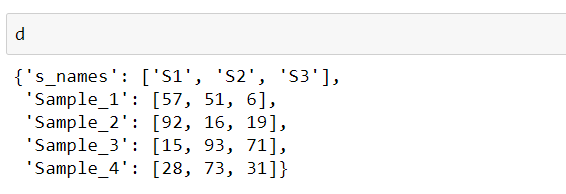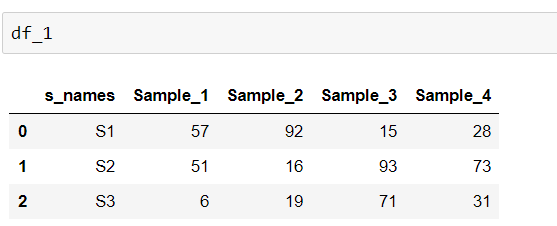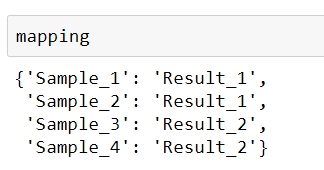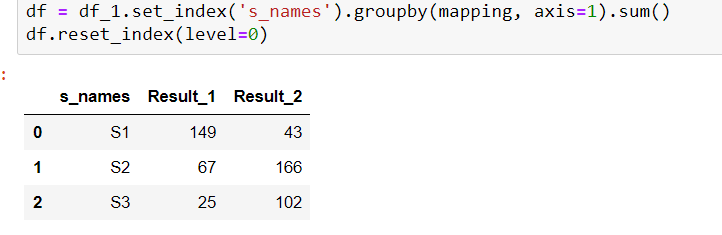Example 2:

 `# Python program to collapse ` `# multiple Columns using Pandas ` `import` `pandas as pd ` `df ``=` `pd.DataFrame({``'First'``: [``'Manan '``, ``'Raghav '``, ``'Sunny '``], ` `                   ``'Last'``: [``'Goel'``, ``'Sharma'``, ``'Chawla'``], ` `                   ``'Age'``:[``12``, ``24``, ``56``]}) ` ` `  `mapping ``=` `{``'First'``: ``'Full Name'``, ``'Last'``: ``'Full Name'``} ` ` `  `df ``=` `df.set_index(``'Age'``).groupby(mapping, axis ``=` `1``).``sum``() ` ` `  `df.reset_index(level ``=` `0``) `

Output: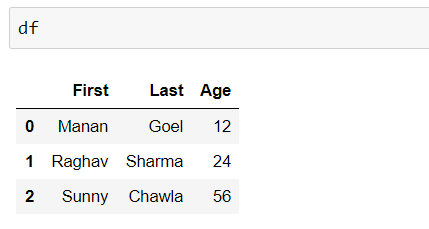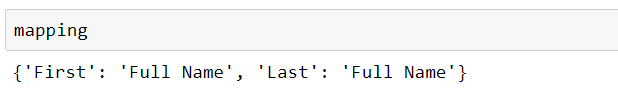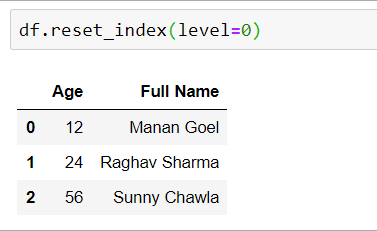My Personal Notes arrow_drop_upIf you like GeeksforGeeks and would like to contribute, you can also write an article using contribute.geeksforgeeks.org or mail your article to contribute@geeksforgeeks.org. See your article appearing on the GeeksforGeeks main page and help other Geeks.

Please Improve this article if you find anything incorrect by clicking on the "Improve Article" button below.

Article Tags :

Be the First to upvote.

Please write to us at contribute@geeksforgeeks.org to report any issue with the above content.